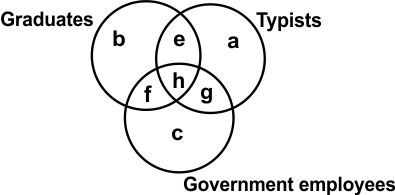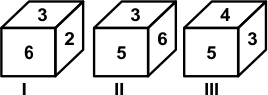﻿ Reasoning Olympiad (CRO) Previous Year Paper for Class 10

# CREST Reasoning Olympiad Class 10 Previous Year Papers

CREST Reasoning Olympiad Previous Year Paper PDF for Class 10:

## Syllabus:

Section 1: Series Completion, Analogy and Classification, Coding - Decoding, Blood Relations, Sequential Output Tracing , Direction Sense Test, Logical Venn Diagrams, Alphabet Test and Logical, Alpha-Numeric, Number, Mathematical Operations, Inserting the Missing Characters, Analytical Reasoning, Mirror Images and Water Images, Spotting Out The Embedded, Figure Matrix, Paper Folding and Paper Cutting, Grouping of Identical Figures, Cubes and Dice, Dot Situation, Construction of Squares and Triangles, Figure Formation and Analysis.

Achievers Section: Higher Order Thinking Questions - Syllabus as per Section 1

 Q.1 The figure given below is made of three circles intersecting one another. These circles represent graduates, typists and government employees. The intersecting regions have been denoted by a, b, c, e, f, g and h, respectively. Study the diagram carefully and answer the question that follows. Which of the following letters represents the typists are only graduates?Q.2 If '+' means '-', '÷' means '+', '-' means '×' and '×' means '÷', then which of the following equation is correct?
 Q.3 In the question given below, there is a statement followed by two conclusions numbered I and II. You have to assume everything in the statement to be true, then consider the two conclusions together and decide which of them logically follows beyond a reasonable doubt from the information given in the statement. Statement- The nation 'X' faced the increased international opposition due to its decision of performing eight nuclear explosions. Conclusions I. The citizens of the nation have favoured the decision.II. Some powerful nations do not want that others may become powerful.
 Q.4 In the following question, choose which number completes the second pair in the same way as the first pair. T/20 : S/19 : : L/12 : ?
 Q.5 Which set of letters completes the second pair in the same way as the first pair? ADJM is to ZWQN, as FKIM is to ___________.
 Q.6 Three positions of a dice are given below. Identify the number on the face opposite to 6.Q.7 In the question given below, there are two statements followed by two conclusions numbered I and II. You have to take the two given statements to be true even if they seem to be at variance from commonly known facts and decide which of the given conclusion(s), logically follow(s) from the two given statements disregarding commonly known facts. StatementsI. Some rats are cats. II. All cats are bats. Conclusions I. No rats are cats.II. Some rats are bats.
 Q.8 At an office where an interview was conducted to select persons for clerical posts, they came to know that, out of 20 persons, 12 knew only typing and 5 knew only shorthand and the rest knew both typing and shorthand. Which of the following diagrams represents this fact?
 Q.9 Pointing to the man in the photograph, Robert said "His mother has only one grandchild whose mother is my sister." How is Robert related to the man in photograph?
 Q.10 Use the relationships defined below to solve the question. 'P × Q' means 'P is the father of Q'. 'P * Q' means 'P is the sister of Q'. 'P + Q' means 'P is the brother of Q'. 'P - Q' means 'P is the mother of Q'. 'P ÷ Q' means 'P is the son of Q'. P = Q' means 'P is the daughter of Q'. If Z × X - Y, then which of the following is true?

 Q.1 a Q.2 c Q.3 b Q.4 b Q.5 b Q.6 b Q.7 b Q.8 c Q.9 b Q.10 b

Preparation is a crucial factor for class 10 children to achieve the greatest possible results in the CREST Reasoning Olympiad exam (CRO). Students who work through the CREST Reasoning Olympiad past year paper for class 10 are able to crack the test with confidence and get to know their weaknesses and have the opportunity to improve upon them before taking their actual exam.

Additionally, students will learn to manage their time more effectively after practicing through the CREST Reasoning Olympiad previous year paper for class 10.